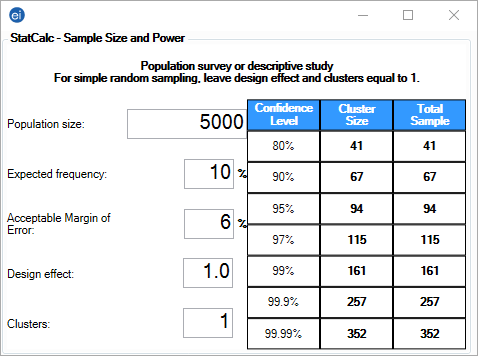# StatCalc: Statistical Calculators

StatCalc

### Population Survey or Descriptive Study

The population survey calculates the sample size recommended for a survey given a set of parameters and the desired confidence level.

#### Guidelines

• The sample must be simple random or representative. A systematic sample (e.g., every fifth person on a list) is acceptable if the sample is representative. Choosing every other person from a list of couples would not give a representative sample because the sample population may include only males or only females.
• The survey questions must have a yes-no or other two-choice answer, leading to a proportion of the population (those answering yes) as the final result.
• For simple random sampling, the values for Design Effect and Clusters are 1 by definition.

#### Example

The following example investigates whether the true prevalence of the HIV antibody in a population is 10%. A random or systematic sample of the population is planned to estimate the prevalence. The desired sample size will be able to identify the prevalence of HIV with a +/- 3% margin of error. The application will show the sample size for several different confidence levels.

1. From the Epi Info™ main page, select StatCalc.
2. Select Population Survey. The Population Survey or Descriptive Study window opens.
3. Enter the Population Size of 5,000.
4. Enter the Expected Frequency of 10%.
5. Enter the Acceptable Margin of Error of 6%.
6. Enter the Design Effect of 1. This input designates the ratio of the variance of responses with clusters to the variance of a simple random sample.
7. Enter the Clusters value of 1. This input designates the number of clusters in the population survey.

The results appear in the window.Figure 10.10: Population Survey or Descriptive Study

The Total Sample column is the product of Cluster Size and Clusters. Each row designates how large of a sample size is required to achieve the necessary confidence level. Sample size determination is only a rough guide. Many other factors (e.g., cost, number of available subjects, rate of nonresponse, and the accuracy of answers and data transcription) must be considered in study design.

Page last reviewed: September 16, 2022, 12:00 pm